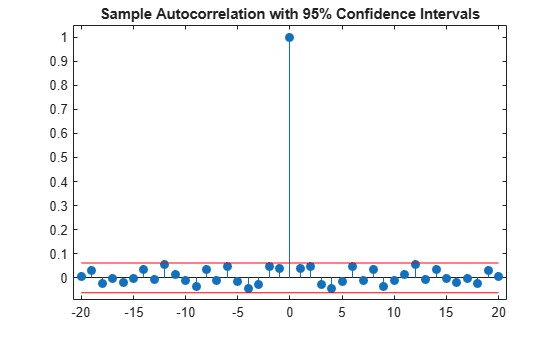# Confidence Intervals for Sample Autocorrelation

This example shows how to create confidence intervals for the autocorrelation sequence of a white noise process. Create a realization of a white noise process with length $L=1000$ samples. Compute the sample autocorrelation to lag 20. Plot the sample autocorrelation along with the approximate 95%-confidence intervals for a white noise process.

Create the white noise random vector. Set the random number generator to the default settings for reproducible results. Obtain the normalized sampled autocorrelation to lag 20.

```rng default L = 1000; x = randn(L,1); [xc,lags] = xcorr(x,20,'coeff');```

Create the lower and upper 95% confidence bounds for the normal distribution $N\left(0,1/L\right)$, whose standard deviation is $1/\sqrt{L}$. For a 95%-confidence interval, the critical value is $\sqrt{2}\phantom{\rule{0.16666666666666666em}{0ex}}{erf}^{-1}\left(0.95\right)\approx 1.96$ and the confidence interval is

`$\Delta =0±\frac{1.96}{\sqrt{L}}.$`

`vcrit = sqrt(2)*erfinv(0.95)`
```vcrit = 1.9600 ```
```lconf = -vcrit/sqrt(L); upconf = vcrit/sqrt(L);```

Plot the sample autocorrelation along with the 95%-confidence interval.

```stem(lags,xc,'filled') hold on plot(lags,[lconf;upconf]*ones(size(lags)),'r') hold off ylim([lconf-0.03 1.05]) title('Sample Autocorrelation with 95% Confidence Intervals')```You see in the above figure that the only autocorrelation value outside of the 95%-confidence interval occurs at lag 0 as expected for a white noise process. Based on this result, you can conclude that the data are a realization of a white noise process.